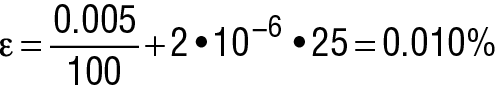Optimize Sense Resistor Cost and Accuracy for RTD Temp Measurement when Using LTC2983 Temp-to-Bits IC

The temperature measurement using a RTD, or a Thermistor, is really a measurement of resistance. RTDs and Thermistors change their resistance with temperature. Once the resistance of the probe is measured, and assuming that the Resistance as a function of temperature is known, the temperature of the probe can be calculated. That function is given by the temperature probe manufacturer, or it can be obtained through characterization. So, and this is the hard part, all one has left to do is to measure resistance of the probe.... accurately.

The LTC2983 performs the resistance measurement in the ratiometric sense. In principle, a known resistance RSENSE is used as a "measuring stick" against which the unknown resistance, RT, of the probe is measured. Therefore the accuracy of the "measuring stick" directly affects the RT measurement, and with that, the accuracy of the temperature measurement. The obvious approach is to use the best possible, "gold standard", shiny "measuring stick". If one such existed it would, most likely, be very expensive... the precision resistors that do exist already are pricey, even though they are not perfect.

So, there is a tradeoff to be considered: how much error resulting from the imperfect RSENSE can my system tolerate? Typically the more error can be tolerated, the less "shiny", the less expensive RSENSE can be used.

But, how does the RSENSE accuracy, or lack thereof, contribute to the overall system error? The following treatment is intended to help with this question.

The LTC2983 computes the resistance RT of the RTD probe from the ratiometric measurement: a current IS is pushed through a stack of resistors RSENSE and RT, the voltages V1 and V2 are simultaneously sensed across the resistors as shown in Figure 1.

Expressing current IS in terms of voltages V1 and V2, and resistances RSENSE and RT, and manipulating the expressions yields:(1)

The calculation in (1) is performed by the LTC2983 automatically. Then the internally stored look up table (LUT) is used to translate RT to temperature readout, T.

In order to evaluate the effect of resistor tolerances on the temperature measurement, express V1 and V2 in terms of I and R. Also, assume that the RTD probe is ideal in its behavior, and the RSENSE resistor deviates from its nominal value by the fraction ε:(2)

Where:

R0 is the resistance of the RTD probe at 0°C

α is the temperature coefficient of the RTD probe

T is the temperature of the RTD probe in °C.

RSENSE is the nominal value of the RSENSE resistor attached to the LTC2983

RSENSE_REG is the programmed value of the RSENSE resistor into the LTC2983 configuration register

ε is the RSENSE resistor error

Typically, the designer sets RSENSE and RSENSE_REG to be equal. Then by using the Taylor series expansion of:(3)

And using this to further simplify the expression for RT:(4)

The expression in (4) gives RT as a function of temperature and the sense resistor error ε. But the typical system specification expresses accuracy in terms of temperature. So, to convert RT to Temperature as measured by the LTC2983, TM, use the linear approximation of the RTD characteristic:(5)

TM is simply equal to temperature of the RTD probe, T, modified by the contribution due to the sense resistor error, ε. Denote that error contribution as a temperature error TE:(6)

Expression (6) enables a systematic approach to trading off the system specification, TE, versus the component specification, ε.

Example

Consider a system for which a desired temperature error bound is 0.25°C. The system uses a PT-1000 RTD probe as a temperature sensor, and the temperature measurement spans 0°C to 150°C. The RTD is connected to the LTC2983 with long leads. The RSENSE is in the same enclosure as the LTC2983, and the temperature inside this enclosure never exceeds 50°C.

Simply put, the system temperature read out error is bounded by:(7)

The equation in (6) can be transformed to yield the sense resistor error bound, as follows:(8)

The nominal resistance of the PT-1000 probe is 1kΩ, and its temperature coefficient α = 0.00385Ω/Ω°C. The maximum temperature of the RTD is T = 150°C. Substituting these values into (8) gives the maximum allowed sense resistor error:(9)

The sense resistor error is dominated by its tolerance, expressed in %, and the temperature coefficient of resistance (TCR), expressed as ppm/°C:(10)

Tolerance and TCR specifications are provided by the resistor manufacturer. ΔT is the deviation of the operating temperature of the resistor from the nominal. Consider a VISHAY FOIL S-series resistor with the following specifications:

RNOMINAL = 1000Ω

Tolerance = ±0.005%

TCR = ±2ppm/°C

The data sheet specifies the resistor nominal value at 25°C. Therefore the sense resistor will experience at most 25°C deviation from nominal temperature while in the enclosure. Then the fractional sense resistor error is at most:(11)

This result is already much better than the error bound stated in (9). But for the sake of completeness, continue on to translate ε to the system error at RTD probe temperature of 150°C:(12)

The result in (12) simply restates the result in (11), but in terms of absolute temperature error. Because the selected RSENSE yields results well within the target temp error of 0.25°C, tolerance and/or TCR specifications can be relaxed, suggesting a less expensive component for RSENSE.